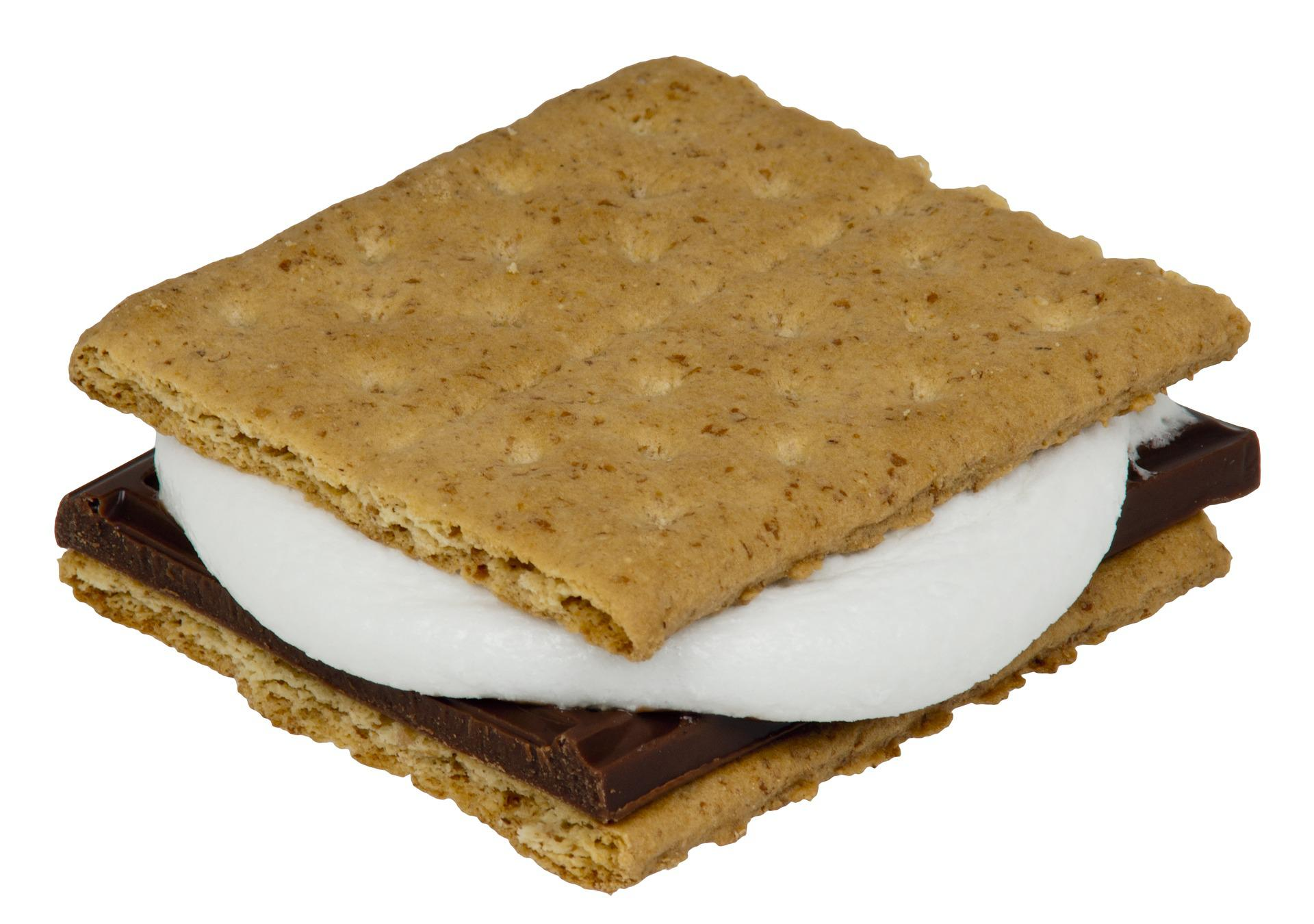# Let’s Do S’more Chemistry!### Introduction

This laboratory activity explores the topics of stoichiometry and limiting reactants in a fun, delicious way! Mass/mole relationships and limiting and excess reactants can be difficult topics for students to grasp. Use this activity to introduce or reinforce these principles.

Stoichiometry is the area of chemistry that allows us to determine the relative quantities of reactants and products in a balanced chemical equation. The law of conservation of mass states that the mass of all participants in a chemical reaction must remain constant over time. Matter is neither created nor destroyed in a chemical reaction, and this principle lays the foundation for stoichiometry.

### Safety

Never allow eating or drinking in a laboratory setting. If you perform this activity with food products, do so only in a dedicated classroom. Making and eating the S’mores is a great way to cap off this activity!

### Procedure

In this basic stoichiometric problem, we’ll assume that 1 S’more represents 1 mole of product. The formula for 1 complete S’more is:

C3G2M
Where C = chocolate rectangle
G = graham cracker square (2 rectangles attached)
M = marshmallow

Have students write a balanced equation for the formation of a complete S’more. This is a good opportunity for you to review pertinent vocabulary (e.g., reactants, products, formulas, coefficients, subscripts) and discuss balancing equations with your students.

3C + 2G + M ? C3G2M

With a balanced equation, students can answer a number of questions about mole-mole and mass-mole relationships using dimensional analysis.

### Student questions

1. Given 30 graham cracker squares, how many S’mores can be made? How many marshmallows and chocolate rectangles do we need to make them?

(30 G) (1 C3G2M / 2 G)  = 15 C3G2M = 15 S’mores
(15 C3G2M) (1 M / 1 C3G2M) = 15 M = 15 Marshmallows needed
(15 C3G2M) (3 C / 1 C3G2M) = 45 C = 45 Chocolate Rectangles needed

2. Suppose you have 34 graham cracker squares, 14 marshmallows, and 40 chocolate rectangles. (A) What is the theoretical yield of S’mores? (B) Which reactant(s) are in excess (left over), and what is the excess for each? (C) Which reactant(s) are the limiting reactant(s) (completely used up)?

(A) Theoretical Yield:
(34 mol G) (1 mol C3G2M / 2 mol G) = 17 S’mores
(14 mol M) (1 mol C3G2M / 1 mol M) = 14 S’mores
(40 mol C) (1 mol C3G2M / 3 mol C) = 13 S’mores
Theoretical yield = 13 moles of S’mores

(B) Excess Reactants:
Graham cracker squares; for 13 S’mores, you will need:
(13 mol C3G2M) (2 mol G / 1 mol C3G2M) = 26 G
34 G – 26 G = 8 moles of G in excess

Marshmallows; for 13 S’mores, you will need:
(13 mol C3G2M) (1 M / 1 mol C3G2M) = 13 G
14 M – 13 M = 1 mole of M in excess
(C) Limiting Reactants:
Chocolate rectangles

3. Given the following theoretical molar mass values for the reactants in the S’mores reaction (see the table below), determine the molar mass of 1 mole of S’mores. This is example data. You could also give students these Food Elements to mass and determine the molar masses themselves.

Examples of molar mass values for S’mores reactants:

 Food Element Molar Mass C, Chocolate Rectangle 3.30 g/mol G, Graham Cracker Square 7.00 g/mol M, Marshmallow 7.10 g/mol

Using the balanced equation above, 1 mole of S’mores has the following molar mass:

3(3.30) + 2(7.00) + 7.10 = 31 g/mol

4. How many S’mores can be made from 100g of each Food Element? Identify any limiting reactants:

100 g Chocolate Rectangles:
(100 g C) (1 mol C / 3.30 g C) (1 mol C3G2M / 3 mol C) = 10.1 mol C3G2M = 10 S’mores100 g Graham Cracker Squares:
(100 g G) (1 mol G / 7.00 g G) (1 mol C3G2M / 2 mol G) = 7.1 mol C3G2M = 7 S’mores100 g Marshmallows:
(100 g M) (1 mol M / 7.10 g M) (1 mol C3G2M / 1 mol M) = 14.1 mol C3G2M = 14 S’mores

In this scenario, Graham Cracker Squares are the limiting reactant, and only 7 S’mores could be made.

5. What is the mass of the S’mores made in question 4?

(7 mol C3G2M) (31 g C3G2M / 1 mol C3G2M) = 217 g C3G2M

You can introduce students to microscale chemistry by making mini S’mores. Simply substitute Golden Grahams cereal for the graham cracker squares, chocolate chips for the chocolate rectangles, and mini marshmallows for the marshmallows. Enjoy!